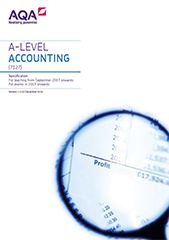# 3.10 Marginal costing

Content

Categorisation of costs by behaviour and understanding of terms.

Costs and terms are:

• direct costs
• indirect costs
• variable costs
• semi-variable costs
• fixed costs
• stepped costs
• marginal cost
• contribution (total and per unit)
• break-even.

Calculation and interpretation of break-even point, interpreting break-even charts and the uses and limitations of break-even analysis methods.

Break-even analysis methods are break-even calculations and break-even charts.

The use of marginal costing in decision making situations.

Decision making will involve the use of marginal costing techniques and consideration of non-financial factors.

Decision making situations are: